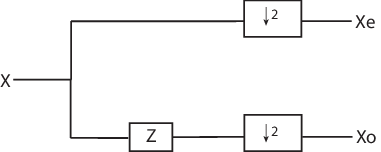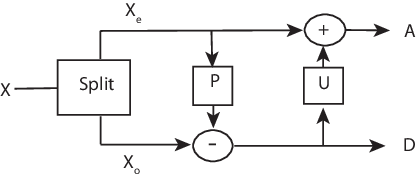## Lifting Method for Constructing Wavelets

The so-called first generation wavelets and scaling functions are dyadic dilations and translates of a single function. Fourier methods play a key role in the design of these wavelets. However, the requirement that the wavelet basis consist of translates and dilates of a single function imposes some constraints that limit the utility of the multiresolution idea at the core of wavelet analysis.

The utility of wavelet methods is extended by the design of second generation wavelets via lifting.

Typical settings where translation and dilation of a single function cannot be used include:

• Designing wavelets on bounded domains — This includes the construction of wavelets on an interval, or bounded domain in a higher-dimensional Euclidean space.

• Weighted wavelets — In certain applications, such as the solution of partial differential equations, wavelets biorthogonal with respect to a weighted inner product are needed.

• Irregularly-spaced data — In many real-world applications, the sampling interval between data samples is not equal.

Designing new first generation wavelets requires expertise in Fourier analysis. The lifting method proposed by Sweldens (see [Swe98] in References) removes the necessity of expertise in Fourier analysis and allows you to generate an infinite number of discrete biorthogonal wavelets starting from an initial one. In addition to generation of first generation wavelets with lifting, the lifting method also enables you to design second generation wavelets, which cannot be designed using Fourier-based methods. With lifting, you can design wavelets that address the shortcomings of the first generation wavelets.

The following section introduces the theory behind lifting, presents the lifting functions of Wavelet Toolbox™ software and gives two short examples:

For more information on lifting, see [Swe98], [Mal98], [StrN96], and [MisMOP03] in References.

### Lifting Background

The DWT implemented by a filter bank is defined by four filters as described in Fast Wavelet Transform (FWT) Algorithm. Two main properties of interest are

• The perfect reconstruction property

• The link with “true” wavelets (how to generate, starting from the filters, orthogonal or biorthogonal bases of the space of the functions of finite energy)

To illustrate the perfect reconstruction property, the following filter bank contains two decomposition filters and two synthesis filters. The decomposition and synthesis filters may constitute a pair of biorthogonal bases or an orthogonal basis. The capital letters denote the Z-transforms of the filters..This leads to the following two conditions for a perfect reconstruction (PR) filter bank:

`$\stackrel{~}{H}\left(z\right)H\left(z\right)+\stackrel{~}{G}\left(z\right)G\left(z\right)=2{z}^{-L+1}$`

and

`$\stackrel{~}{H}\left(-z\right)H\left(z\right)+\stackrel{~}{G}\left(-z\right)G\left(z\right)=0$`

The first condition is usually (incorrectly) called the perfect reconstruction condition and the second is the anti-aliasing condition.

The z–L+1 term implies that perfect reconstruction is achieved up to a delay of one sample less than the filter length, L. This results if the analysis filters are shifted to be causal.

Lifting designs perfect reconstruction filter banks by beginning from the basic nature of the wavelet transform. Wavelet transforms build sparse representations by exploiting the correlation inherent in most real world data. For example, plot the example of electricity consumption over a 3-day period.

```load leleccum; plot(leleccum) grid on; axis tight;```The data do not exhibit arbitrary changes from sample to sample. Neighboring samples exhibit correlation. A relatively low (high) value at index (sample) n is associated with a relatively low (high) value at index n-1 and n+1. This implies that if you have only the odd or even samples from the data, you can predict the even or odd samples. How accurate your prediction is obviously depends on the nature of the correlation between adjacent samples and how closely your predictor approximates that correlation.

### Polyphase Representation

The polyphase representation of a signal is an important concept in lifting. You can view each signal as consisting of phases, which consist of taking every N-th sample beginning with some index. For example, if you index a time series from n=0 and take every other sample starting at n=0, you extract the even samples. If you take every other sample starting from n=1, you extract the odd samples. These are the even and odd polyphase components of the data. Because your increment between samples is 2, there are only two phases. If you increased your increment to 4, you can extract 4 phases. For lifting, it is sufficient to concentrate on the even and odd polyphase components. The following diagram illustrates this operation for an input signal.where Z denotes the unit advance operator and the downward arrow with the number 2 represents downsampling by two. In the language of lifting, the operation of separating an input signal into even and odd components is known as the split operation, or the lazy wavelet.

To understand lifting mathematically, it is necessary to understand the z-domain representation of the even and odd polyphase components.

The z-transform of the even polyphase component is

`${X}_{0}\left(z\right)=\sum _{n}x\left(2n\right){z}^{-n}$`

The z-transform of the odd polyphase component is

`${X}_{1}\left(z\right)=\sum _{n}x\left(2n+1\right){z}^{-n}$`

You can write the z-transform of the input signal as the sum of dilated versions of the z-transforms of the polyphase components.

`$X\left(z\right)=\sum _{n}x\left(2n\right){z}^{-2n}+\sum _{n}x\left(2n+1\right){z}^{-2n+1}={X}_{0}\left({z}^{2}\right)+{z}^{-1}{X}_{1}\left({z}^{2}\right)$`

### Split, Predict, and Update

A single lifting step can be described by the following three basic operations:

• Split — the signal into disjoint components. A common way to do this is to extract the even and odd polyphase components explained in Polyphase Representation. This is also known as the lazy wavelet.

• Predict — the odd polyphase component based on a linear combination of samples of the even polyphase component. The samples of the odd polyphase component are replaced by the difference between the odd polyphase component and the predicted value. The predict operation is also referred to as the dual lifting step.

• Update — the even polyphase component based on a linear combination of difference samples obtained from the predict step. The update step is also referred to as the primal lifting step.

In practice, a normalization is incorporated for both the primal and dual liftings.

The following diagram illustrates one lifting step.### Haar Wavelet Via Lifting

Using the operations defined in Split, Predict, and Update, you can implement the Haar wavelet via lifting.

• Split — Divide the signal into even and odd polyphase components.

• Predict — Replace x(2n+1) with d(n)=x(2n+1)x(2n). The predict operator is simply x(2n).

• Update — Replace x(2n) with x(2n)+d(n)/2. This is equal to (x(2n)+x(2n+1))/2.

The dual lifting in the z domain can be written in the following matrix form

`$\left(\begin{array}{cc}1& 0\\ -P\left(z\right)& 1\end{array}\right)\left(\begin{array}{c}{X}_{0}\left(z\right)\\ {X}_{1}\left(z\right)\end{array}\right)$`

with P(z)=1.

The primal lifting can be written in the z domain in the following matrix form

`$\left(\begin{array}{cc}1& S\left(z\right)\\ 0& 1\end{array}\right)\left(\begin{array}{cc}1& 0\\ -P\left(z\right)& 1\end{array}\right)\left(\begin{array}{c}{X}_{0}\left(z\right)\\ {X}_{1}\left(z\right)\end{array}\right)$`

with S(z)=1/2.

Finally, the primal and dual normalization can be incorporated as follows.

`$\left(\begin{array}{cc}\sqrt{2}& 0\\ 0& \frac{1}{\sqrt{2}}\end{array}\right)\left(\begin{array}{cc}1& S\left(z\right)\\ 0& 1\end{array}\right)\left(\begin{array}{cc}1& 0\\ -P\left(z\right)& 1\end{array}\right)\left(\begin{array}{c}{X}_{0}\left(z\right)\\ {X}_{1}\left(z\right)\end{array}\right)$`

To construct this lifting step in MATLAB®, enter:

```LiftHaar = liftwave('haar'); displs(LiftHaar)```

The following is displayed in the MATLAB command window.

```LiftHaar = {... 'd' [ -1.00000000]  'p' [ 0.50000000]  [ 1.41421356] [ 0.70710678] [] }; ```

`'d'` denotes the dual lifting. Note that for convenience, the negative sign is incorporated into the dual lifting step in the Wavelet Toolbox software. `'p'` denotes the primal lifting and `[ 1.41421356] [ 0.70710678]` are the primal and dual normalization constants. `LiftHaar{1,3}` and `LiftHaar{2,3}` give the highest degree of the Laurent polynomials, which describe the dual and primal liftings. In this case, both are zero because the dual and primal liftings are both described by scalars.

### Bior2.2 Wavelet Via Lifting

This example presents the lifting scheme for the `bior2.2` biorthogonal scaling and wavelet filters.

In the Haar lifting scheme, the dual lifting (predict operator) differenced the odd and even samples. In this example, define a new predict operator that computes the average of the two neighboring even samples. Subtract the average from the intervening odd sample.

`$d\left(n\right)=x\left(2n+1\right)-\frac{1}{2}\left[x\left(2n\right)+x\left(2n+2\right)\right]$`

In the z-domain you can write the dual lifting step as

`$\left(\begin{array}{cc}1& 0\\ -\frac{1}{2}\left(1+z\right)& 1\end{array}\right)\left(\begin{array}{c}{X}_{0}\left(z\right)\\ {X}_{1}\left(z\right)\end{array}\right)$`

To obtain the primal lifting, or update, examine the primal lifting in Haar Wavelet Via Lifting. The update is defined in such a way that the sum of the approximation coefficients is proportional to the mean of the input data vector.

`$\sum _{n}x\left(n\right)=\frac{1}{2}\sum _{n}a\left(n\right)$`

To obtain the same result in this lifting step, define the update as

`$\left(\begin{array}{cc}1& \frac{1}{4}\left({z}^{-1}+1\right)\\ 0& 1\end{array}\right)\left(\begin{array}{cc}1& 0\\ -\frac{1}{2}\left(1+z\right)& 1\end{array}\right)\left(\begin{array}{c}{X}_{0}\left(z\right)\\ {X}_{1}\left(z\right)\end{array}\right)$`

To obtain this lifting scheme at the command line, enter:

`liftwave('bior2.2')`

### Lifting Functions

The lifting functions of the toolbox are organized into five groups:

#### Lifting Schemes

Function Name

Description

`lsinfo`

`displs`

Display a lifting scheme

`addlift`

Add primal or dual elementary lifting steps to a lifting scheme

`wavenames`

Wavelets with lifting schemes

#### Biorthogonal Quadruplets of Filters and Lifting Schemes

These functions connect lifting schemes to biorthogonal quadruplets of filters and associated scaling and wavelet function pairs.

Function Name

Description

`liftfilt`

Apply elementary lifting steps on quadruplet of filters

`filt2ls`

Transform a quadruplet of filters to a lifting scheme

`ls2filt`

Transform a lifting scheme to a quadruplet of filters

`bswfun`

Compute and plot biorthogonal “scaling and wavelet” functions

These functions provide some basic lifting schemes associated with some usual orthogonal or biorthogonal (“true”) wavelets and the “lazy” one. These schemes can be used to initialize a lifting procedure.

Function Name

Description

`wavenames`

Provides usual wavelet names available for LWT

`liftwave`

Provides lifting scheme associated with a usual wavelet

`wave2lp`

Provides Laurent polynomials associated with a usual wavelet

#### Lifting Wavelet Transform (LWT)

These functions contain the direct and inverse lifting wavelet transform (LWT) files for both 1-D and 2-D signals. LWT reduces to the polyphase version of the DWT algorithm with zero-padding extension mode and without extra-coefficients.

Function Name

Description

`lwt`

1-D lifting wavelet transform

`ilwt`

Inverse 1-D lifting wavelet transform

`lwtcoef`

Extract or reconstruct 1-D LWT wavelet coefficients

`lwt2`

2-D lifting wavelet transform

`ilwt2`

Inverse 2-D lifting wavelet transform

`lwtcoef2`

Extract or reconstruct 2-D LWT wavelet coefficients

#### Laurent Polynomials and Matrices

These functions permit an entry to representation and calculus of Laurent polynomials and matrices.

Function Name

Description

`laurpoly`

Constructor for the class of Laurent polynomials

`laurmat`

Constructor for the class of Laurent matrices

The lifting folder and the two object folders `@laurpoly` and `@laurmat` contain many other files.

### Primal Lifting from Haar

These two simple examples illustrate the basic lifting capabilities of Wavelet Toolbox software.

A primal lifting starting from Haar wavelet.

Start from the Haar wavelet and get the corresponding lifting scheme.

```lshaar = liftwave('haar'); displs(lshaar);```

Add a primal ELS to the lifting scheme.

```els = {'p',[-0.125 0.125],0}; lsnew = addlift(lshaar,els); displs(lsnew);```

Transform the lifting scheme to biorthogonal filters quadruplet and plot the resulting scaling function and wavelet.

```[LoD,HiD,LoR,HiR] = ls2filt(lsnew); bswfun(LoD,HiD,LoR,HiR,'plot');```### Integer-to-Integer Wavelet Transform

In several applications it is desirable to have a wavelet transform that maps integer inputs to integer scaling and wavelet coefficients. You can accomplish easily using lifting.

Start with the Haar transform for an integer to integer wavelet transform and apply a primal lifting step.

```lshaar = liftwave('haar','int2int'); els = {'p',[-0.125 0.125],0}; lsnewint = addlift(lshaar,els);```

Obtain the integer-to-integer wavelet transform of a 1-D signal and invert the transform to demonstrate perfect reconstruction.

```x = 1:8; [cA,cD] = lwt(x,lsnewint); xnew = ilwt(cA,cD,lsnewint)```

## Support평가판 신청